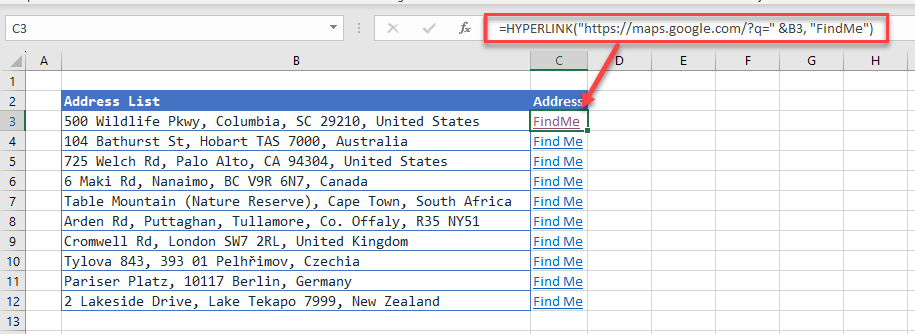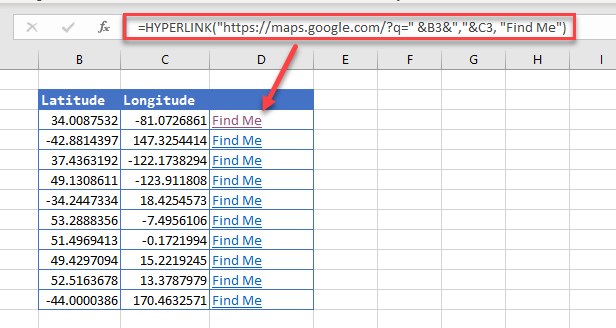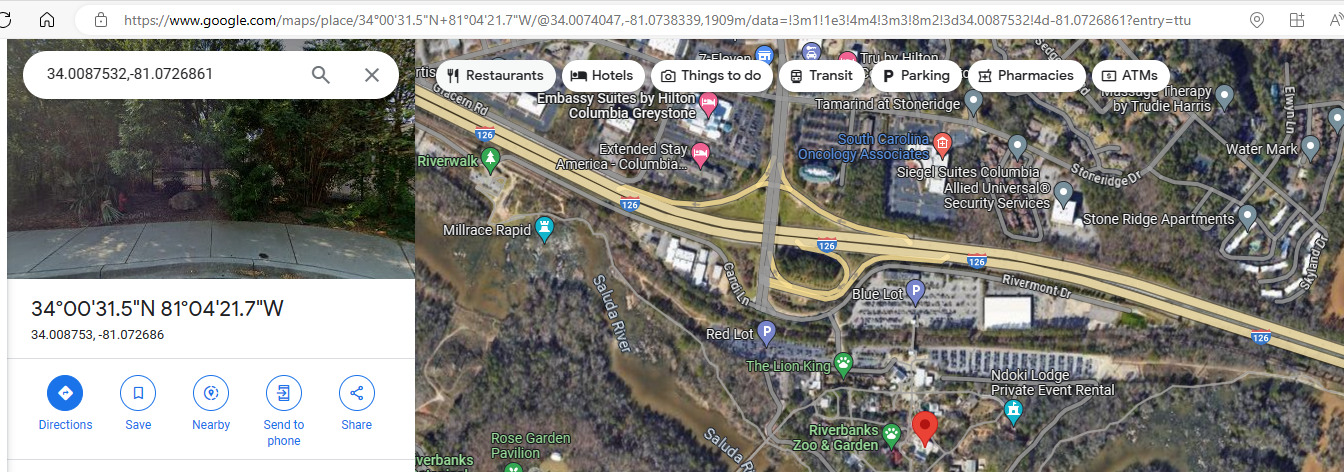## Build a Custom URL for Google Maps

If you have a list of addresses stored in Excel, you can create a hyperlink in an adjacent cell which will open Google Maps and navigate to the address.

`=HYPERLINK("https://maps.google.com/?q=" &[celladdress], "Find Me")`

where [celladdress] is equal to the cell where the address is stored and the “Find Me” is the text that will be displayed on your Excel sheet.

## Use Latitude and Longitude for Google Maps

If you do not have the physical address, but you have the map co-ordinates, you can build these into your hyperlink.

The hyperlink changes slightly such as the example below:

`=HYPERLINK("https://maps.google.com/?q=" &[latitude]&","&[longitude], "Find Me")`

where [latitude] is the cell address of the latitude co-ordinate and [longitude] is the cell address of the longitude co-ordinate.## Show the Satellite Map with Google Maps

If we adjust the formula slightly, we will see the satellite image of the map:

```=HYPERLINK("https://maps.google.com/?t=k&q=" &B3&","&C3, "Find Me")```